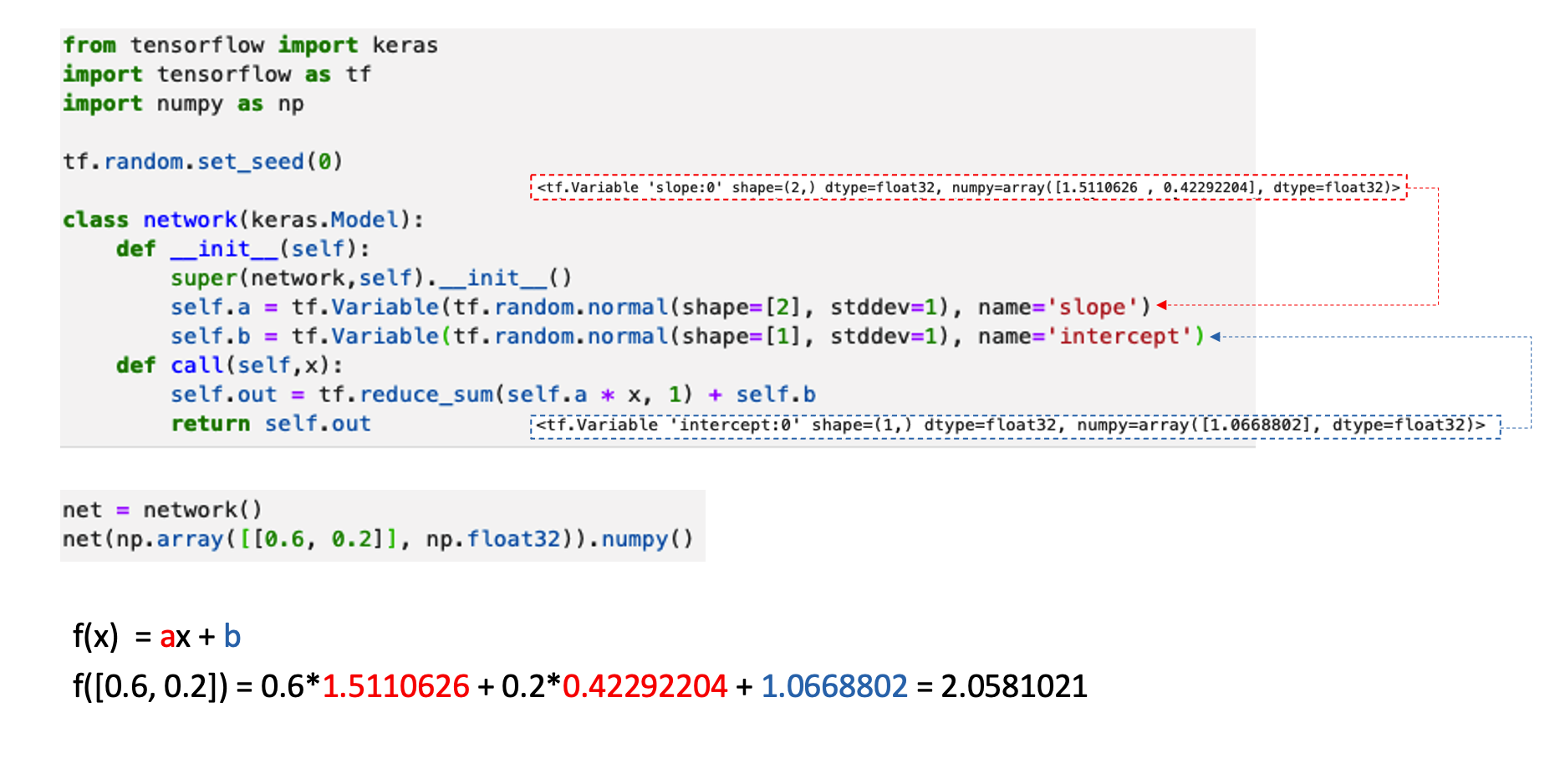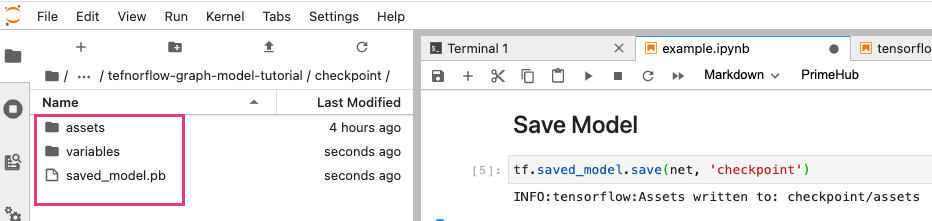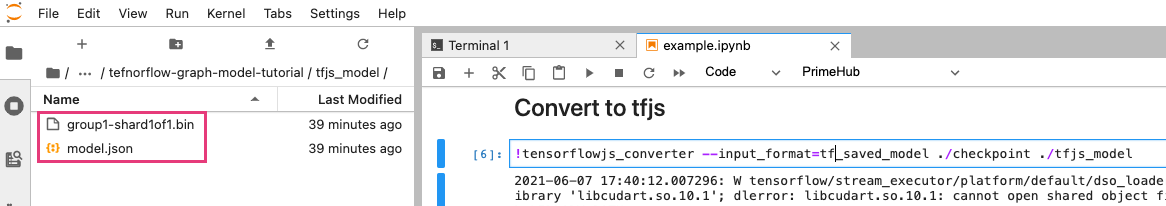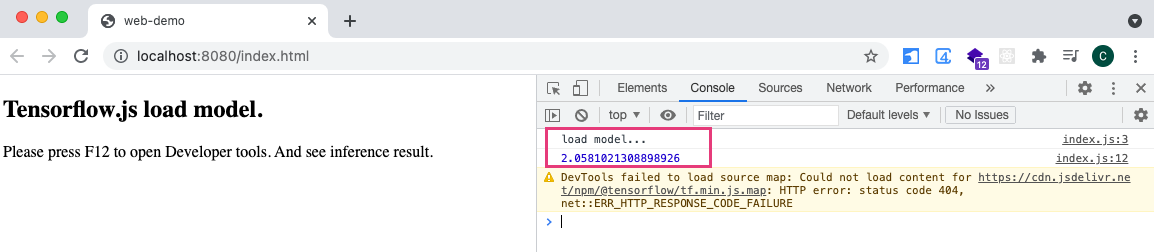# Python TensorFlow2 儲存自定義 Graph 模型並轉成 tfjs

2021/06/07

## 建立網路

``````from tensorflow import keras
import tensorflow as tf
import numpy as np

tf.random.set_seed(0)

class network(keras.Model):
def __init__(self):
super(network,self).__init__()
self.a = tf.Variable(tf.random.normal(shape=, stddev=1), name='slope')
self.b = tf.Variable(tf.random.normal(shape=, stddev=1), name='intercept')
def call(self,x):
self.out = tf.reduce_sum(self.a * x, 1) + self.b
return self.out
``````

``````net = network()
net(np.array([[0.6, 0.2]], np.float32)).numpy()
``````## 儲存模型

``````tf.saved_model.save(net, 'checkpoint')
``````## 載入模型與測試

``````net_1 = tf.saved_model.load('checkpoint')
net_1(np.array([a[0.6, 0.2]], np.float32)).numpy()
``````
``````# output
array([2.0581021], dtype=float32)
``````

## 轉換成 tensorflowjs

``````pip install tensorflowjs
``````

• `--input_format=tf_saved_model`
• 指定欲轉換的模型格式
• 後面兩個路徑分別為
• 欲轉換的模型資料夾
• 轉換 tfjs 後的存放路徑
``````tensorflowjs_converter --input_format=tf_saved_model ./checkpoint ./tfjs_model
``````## 前端網頁載入 TensorFlow 模型

### 載入 CDN

``````<!DOCTYPE html>
<html lang="en">
<meta charset="UTF-8">
<meta http-equiv="X-UA-Compatible" content="IE=edge">
<meta name="viewport" content="width=device-width, initial-scale=1.0">
<title>web-demo</title>

Please press F12 to open Developer tools. And see inference result.

<script src="https://cdn.jsdelivr.net/npm/@tensorflow/tfjs@3.7.0"></script>
<script src="./js/index.js"></script>
</body>
</html>
``````

### 前端 JavaScript

``````async function init(){
predict();
}

function predict(){
let tensor=tf.tensor([[0.6, 0.2]]);
// 預測
const pred = model.predict(tensor);
const result = pred.dataSync();
console.log(result)
}
``````https://www.youtube.com/channel/UCSNPCGvMYEV-yIXAVt3FA5A